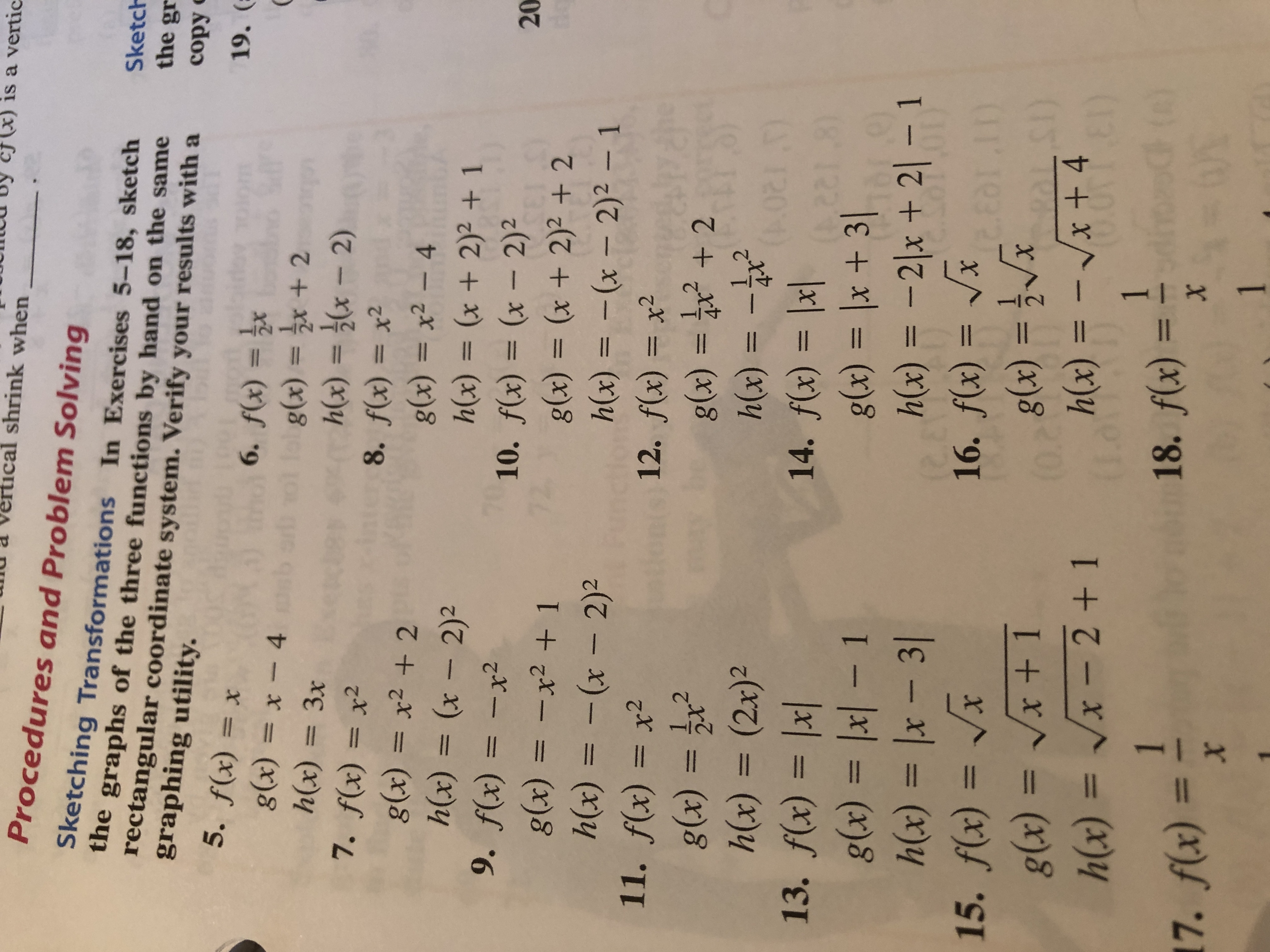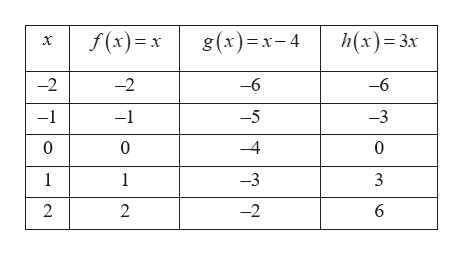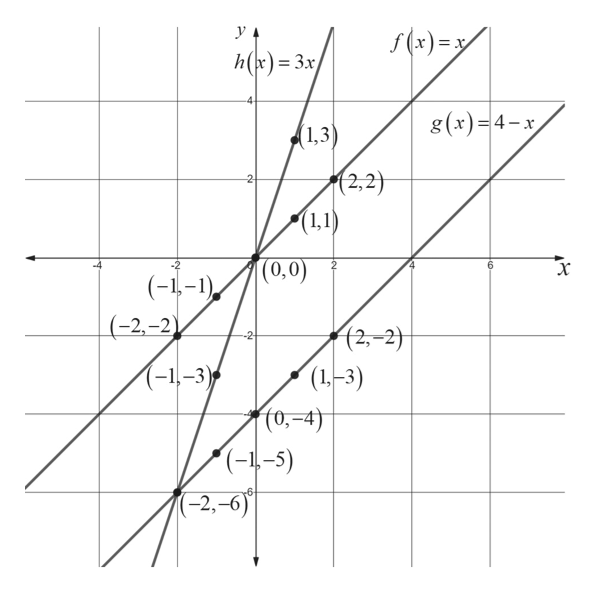# is a verticshrink whenProcedures and Problem SolvingSketching Transformations In Exercises 5-18, sketchthe graphs of the three functions by hand on the samerectangular coordinate system. Verify your results with agraphing utility.Sketchthe grcopy19. (5. f(x) x6. f(x) = xfobg(x) = 2x +2h(x) (x-2)g(x) = x-4h(x) = 3x7. f(x)= x2g(x)= x2 +2h(x) (x - 2)29. f(x)=-x28. f(x) = x2g(x) = x2 - 4h(x) = (x + 2)2 +10. f(x) = (x - 2)g(x) = (x + 2)2 + 2h(x) = -(x 2)2 - 112. f(x) x2g(x) = +2h(x) =20g(x) =-x2 +h(x) = -(x - 2)211. f(x) = x212111.24Xh(x) = (2x)214. f(x) = lx13. f(x)= lx|g(x) = |x| - 1h(x) = lx - 3115. f(x) xg(x) = Vx + 1h(x) = Vx - 2 +1,281g(x) = lx + 31h(x) = -2|x + 21 -116. f(x) = VxOTITD)h(x) = -Vx + 4(08() =118. f(x)X117. f(x)X1

Question
1 viewshelp_outlineImage Transcriptioncloseis a vertic shrink when Procedures and Problem Solving Sketching Transformations In Exercises 5-18, sketch the graphs of the three functions by hand on the same rectangular coordinate system. Verify your results with a graphing utility. Sketch the gr copy 19. ( 5. f(x) x 6. f(x) = x fobg(x) = 2x +2 h(x) (x-2) g(x) = x-4 h(x) = 3x 7. f(x)= x2 g(x)= x2 +2 h(x) (x - 2)2 9. f(x)=-x2 8. f(x) = x2 g(x) = x2 - 4 h(x) = (x + 2)2 + 10. f(x) = (x - 2) g(x) = (x + 2)2 + 2 h(x) = -(x 2)2 - 1 12. f(x) x2 g(x) = +2 h(x) = 20 g(x) =-x2 + h(x) = -(x - 2)2 11. f(x) = x2 12 11 1.2 4X h(x) = (2x)2 14. f(x) = lx 13. f(x)= lx| g(x) = |x| - 1 h(x) = lx - 31 15. f(x) x g(x) = Vx + 1 h(x) = Vx - 2 +1 ,281 g(x) = lx + 31 h(x) = -2|x + 21 -1 16. f(x) = Vx OTIT D) h(x) = -Vx + 4 (08() = 1 18. f(x) X 1 17. f(x) X 1 fullscreen
check_circle

Step 1

Hey, since there are multiple questions posted, we will answer first question(5 th question). If you want any specific question to be answered then please submit that question only or specify the question number in your message.

The given functions are,

Step 2

Tabulate the functional values as shown below.help_outlineImage Transcriptionclosef(x) g(x)x-4 h(x) 3x -2 -2 -6 -6 -1 -1 -5 -3 0 0 -4 0 1 1 -3 3 2 2 -2 6 T fullscreen
Step 3

Plot the above points and obtain the graphs of...help_outlineImage Transcriptionclosey f(x)= h(r) = 3x/ g(x)=4-x 1,3 (2,2) 2 (1,1) |(0,0) X 6 (--1) (-2,-2) (2,-2) (1,-3) f0,-4) (-1-5) (-1,-3) (-2,-6) fullscreen

### Want to see the full answer?

See Solution

#### Want to see this answer and more?

Solutions are written by subject experts who are available 24/7. Questions are typically answered within 1 hour.*

See Solution
*Response times may vary by subject and question.
Tagged in

### Other